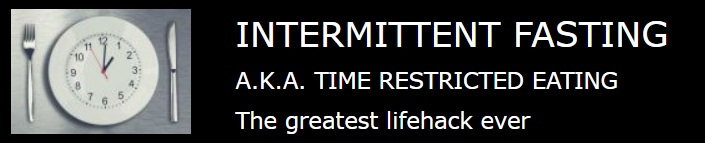# Kotlin: Persistent Chronometer, keeps counting after restart

Topics interesting only for Kotlin / Android / SQLite programmers

STEPS:

@ Create object AppPrefs as described here. It allows to store LocalDateTime values in the application's SharedPreferences in a convenient way (we need to remember the moment when the Chronometer started - that moment will be used to calculate the new base when the Chronometer's parent Activity is resuming).

@ In "util" package, created in first step, create a Kotlin file named ExtensionFunctions (if you don't have it) and add the following code to it:

Code: Select all
`import android.content.Contextimport android.os.SystemClockimport android.widget.Chronometerimport java.time.Durationimport java.time.LocalDateTime// -----------------------------------------------------------------------------------------------------------------// ------- Extend Chronometer functions to allow persistence (http://code.intfast.ca/viewtopic.php?t=826):// -----------------------------------------------------------------------------------------------------------------// The key, by which the base time of the Chronometer (as a LocalDateTime) will be stored in SharedPreferences,// to be then retrieved onResume() and used to calculate the new base to make the Chronometer keeping counting:fun Chronometer.generateStartLdtPrefKey(instanceName: String?): String =                                        "-+=|[Chronometer \${instanceName ?: ""} START LDT]|=+-" // LDT = LocalDateTimefun Chronometer.start(instanceName: String? = null /* omit if only one Chronometer in app */, context: Context) {    // Must be called instead of start(<no args>) when user clicks START button.    // Starts Chronometer and makes it counting from the curr. moment (now).    // To count form a provided moment, use another overload.    // Remember the curr. moment, so resume(), called from the Activity onResume(), will make the Chronometer counting from it:    val startLdtPrefKey = this.generateStartLdtPrefKey(instanceName ?: "")    AppPrefs.put(startLdtPrefKey, LocalDateTime.now()!!, context)    this.base = SystemClock.elapsedRealtime() // milliseconds since boot, including time spent in sleep    this.start()} // Chronometer.start(String, Context)fun Chronometer.start(instanceName: String? = null /* omit if only one Chronometer in app */,                      startLdt: LocalDateTime? /* null = "start from now" */, context: Context) {    // Starts Chronometer and makes it counting from the provided moment (startLdt).    // To count form the curr. moment (now), use another overload.    if (startLdt == null) {        start(instanceName, context) // count form the curr. moment (now)        return    }    // Remember that moment, so resume(), called from the Activity onResume(), will make the Chronometer counting from it:    val startLdtPrefKey = generateStartLdtPrefKey(instanceName)    AppPrefs.put(startLdtPrefKey, startLdt, context)    val now = LocalDateTime.now()!!    var deltaInMilli = Duration.between(startLdt, now)!!.toMillis()    val secondsInMilli = 1000L    val minutesInMilli = secondsInMilli * 60    val hoursInMilli = minutesInMilli * 60    val daysInMilli = hoursInMilli * 24    deltaInMilli %= daysInMilli    val elapsedHours = deltaInMilli / hoursInMilli    deltaInMilli %= hoursInMilli    val elapsedMinutes = deltaInMilli / minutesInMilli    deltaInMilli %= minutesInMilli    val elapsedSeconds = deltaInMilli / secondsInMilli    val elapsedTimeMilliseconds =        elapsedHours * 60 * 60 * 1000 + elapsedMinutes * 60 * 1000 + elapsedSeconds * 1000    this.base = SystemClock.elapsedRealtime() - elapsedTimeMilliseconds    this.start()} // Chronometer.start(String, LocalDateTime, Context)fun Chronometer.finish() {    // Must be called instead of stop() when user clicks STOP button.    this.stop()    this.base = SystemClock.elapsedRealtime() // reset displayed time to "00:00"} // Chronometer.finish()fun Chronometer.resume(instanceName: String? = null /* omit if only one Chronometer in app */, context: Context) {    // Must be called from onResume() of the Chronometer's parent Activity    val startLdtPrefKey = generateStartLdtPrefKey(instanceName)    val startLdt = AppPrefs.getLocalDateTime(startLdtPrefKey, context)        ?:        return // it's null because start(context: Context) has never been called    start(instanceName, startLdt, context)} // resume()`

@ Create the Chronometer on the Activity. Give it a name (ID). Let's say, you called it myChronometer.

@ Write in the function which starts myChronometer (for example, when user clicks the START button):

Code: Select all
`myChronometer.start(this)`

@ Write in the function which stops myChronometer (for example, when user clicks the STOP button):

Code: Select all
`myChronometer.finish(this)`

@ Write in onResume() of myChronometer's parent Activity (that's where the magic happens!):

Code: Select all
`myChronometer.resume(this)`

@ If you want to listen to time changes in your Chronometer (i.e. to do some action when some time has been achieved), do these steps.

IF YOU HAVE MORE THAN ONE CHRONOMETER IN YOUR APPLICATION...

...then ALWAYS pass the chronometer name (i.e. the name of the pointer variable) to start() and resume(). Internally, that name will be used as the part of the key, by which the start time of each Chronometer is stored in the SharedPreferences

Code: Select all
`firstChronometer.start("firstChronometer", this)secondChronometer.start("secondChronometer", this)...firstChronometer.resume("firstChronometer", this)secondChronometer.resume("secondChronometer", this)`

Pay attention, that if you have more than one Chronometer in your app, you must give them names (IDs) unique per app - even if they are placed on different Activities. If you want to give them a same name, then pass to start() and resume() other strings, which identify each Chronometer (like "ActivityName.ChronometerName").Ursego

Posts: 143
Joined: 19 Feb 2013, 20:33

## IF you want to ((lose weight) OR (have unbelievable (brain function AND mental clarity))) THEN click:Traffic Counter